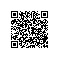# 输入任意两正整数，获取该区间所有素数


from math import sqrt

from pip._vendor.distlib.compat import raw_input

start = int(raw_input('请输入开始正整数 ： '))
end = int(raw_input('请输入结束正整数 ： '))
h = 0
leap = 1
for m in range(start+1,end+1):
k = int(sqrt(m + 1))
for i in range(2,k + 1):
if m % i == 0:
leap = 0
break
if leap == 1:
print ('%-4d' % m)
h += 1
if h % 10 == 0:
print ('')
leap = 1
print ('The total is %d' % h)使用钉钉扫一扫加入圈子
+ 订阅